GMAT  >  Test: Exponents- 3

# Test: Exponents- 3

Test Description

## 15 Questions MCQ Test General Aptitude for GATE | Test: Exponents- 3

Test: Exponents- 3 for GMAT 2022 is part of General Aptitude for GATE preparation. The Test: Exponents- 3 questions and answers have been prepared according to the GMAT exam syllabus.The Test: Exponents- 3 MCQs are made for GMAT 2022 Exam. Find important definitions, questions, notes, meanings, examples, exercises, MCQs and online tests for Test: Exponents- 3 below.
Solutions of Test: Exponents- 3 questions in English are available as part of our General Aptitude for GATE for GMAT & Test: Exponents- 3 solutions in Hindi for General Aptitude for GATE course. Download more important topics, notes, lectures and mock test series for GMAT Exam by signing up for free. Attempt Test: Exponents- 3 | 15 questions in 15 minutes | Mock test for GMAT preparation | Free important questions MCQ to study General Aptitude for GATE for GMAT Exam | Download free PDF with solutions
 1 Crore+ students have signed up on EduRev. Have you?
Test: Exponents- 3 - Question 1

###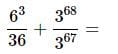Detailed Solution for Test: Exponents- 3 - Question 1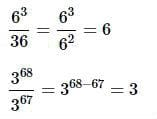Putting these together,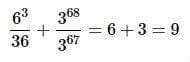Test: Exponents- 3 - Question 2

###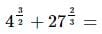Detailed Solution for Test: Exponents- 3 - Question 2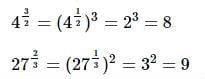Then putting them together,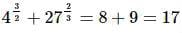Test: Exponents- 3 - Question 3

### Which of the following expressions is equivalent to this expression?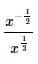You may assume that x>0.

Detailed Solution for Test: Exponents- 3 - Question 3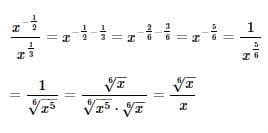Test: Exponents- 3 - Question 4

Simplify the following expression without a calculator: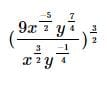Detailed Solution for Test: Exponents- 3 - Question 4

The easiest way to simplify is to work from the inside out. We should first get rid of the negatives in the exponents. Remember that variables with negative exponents are equal to the inverse of the expression with the opposite sign. For example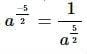So using this, we simplify: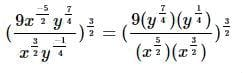Now when we multiply variables with exponents, to combine them, we add the exponents together. For example,  (a2)(a2)=a2+2=a4 Doing this to our expression we get it simplified to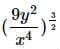The next step is taking the inside expression and exponentiating it. When taking an exponent of a variable with an exponent, we actually multiply the exponents. For example,  (a2)4=a(2∗4)=a8.
The other rule we must know that is an exponent of one half is the same as taking the square root. So for the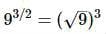So using these rules,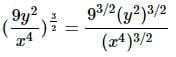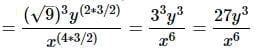Test: Exponents- 3 - Question 5

Rewrite as a single logarithmic expression:

3+log3x−2log3y

Detailed Solution for Test: Exponents- 3 - Question 5

First, write each expression as a base 3 logarithm:
3=log327 since 33=27
2log3y=log3y2
Rewrite the expression accordingly, and apply the logarithm sum and difference rules:
3+log3x−2log3y
=log327+log3x−log3y2

=log3(27⋅x)−log3y2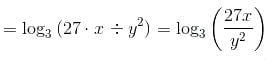Test: Exponents- 3 - Question 6

If 3p+3p+3p=3q, what is p in terms of q?

Detailed Solution for Test: Exponents- 3 - Question 6

We have 3p+3p+3p=3∗3p=3p+1=3q.

So p+1=q, and p=q−1.

Test: Exponents- 3 - Question 7

What are the last two digits, in order, of 6789 ?

Detailed Solution for Test: Exponents- 3 - Question 7

Inspect the first few powers of 6; a pattern emerges.

61=6

62=36

63=216

64=1,296

65=7,776

66=46,656

67=279,936

68=1,679,616

69=10,077,696

610=60,466,176

As you can see, the last two digits repeat in a cycle of 5.

789 divided by 5 yields a remainder of 4; the pattern that becomes apparent in the above list is that if the exponent divided by 5 yields a remainder of 4, then the power ends in the diigts 96.

Test: Exponents- 3 - Question 8

Which of the following expressions is equal to the expression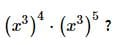Detailed Solution for Test: Exponents- 3 - Question 8

Use the properties of exponents as follows:

(x3)4⋅(x3)5

=x3⋅4 ⋅ x3⋅5

=x12⋅ x15

=x12+15

=x27

Test: Exponents- 3 - Question 9

Simplify: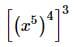Detailed Solution for Test: Exponents- 3 - Question 9

Apply the power of a power principle twice by multiplying exponents: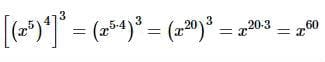Test: Exponents- 3 - Question 10

Divide:
(56x3+21x2−63x+77)÷7x2

Detailed Solution for Test: Exponents- 3 - Question 10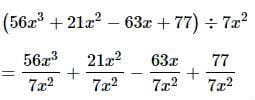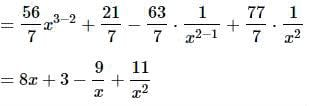Test: Exponents- 3 - Question 11

Solve for N: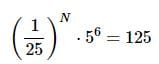Detailed Solution for Test: Exponents- 3 - Question 11

(1/25)⋅ 56=125

(5−2)⋅ 56=53

5−2⋅N ⋅ 56=53

5−2N + 6=53

−2N+6=3

−2N+6−6=3−6

−2N=−3

−2N÷(−2)=−3÷(−2)

N=3/2

Test: Exponents- 3 - Question 12

Which of the following is equal to log8+log5−log4 ?

Detailed Solution for Test: Exponents- 3 - Question 12

log8+log5−log4
=log(8⋅5)−log4
=log40−log4
=log(40÷4)
=log10=1

Test: Exponents- 3 - Question 13

Which of the following is equal to 4log3−2log6 ?

Detailed Solution for Test: Exponents- 3 - Question 13

4log3−2log6

=log(34)−log(62)

=log81−log36

=log81/36

=log9/4

=log 9− log 4

Test: Exponents- 3 - Question 14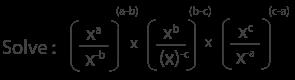Detailed Solution for Test: Exponents- 3 - Question 14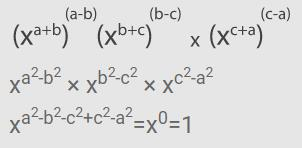Test: Exponents- 3 - Question 15

Which of the following is true about  log51,000 ?

Detailed Solution for Test: Exponents- 3 - Question 15

54=625;55=3,125

Therefore,

log625=4;log3,125=5

log5625<log51,000<log53,125

4<log51,000<5

## General Aptitude for GATE

61 videos|68 docs|88 tests
 Use Code STAYHOME200 and get INR 200 additional OFF Use Coupon Code
Information about Test: Exponents- 3 Page
In this test you can find the Exam questions for Test: Exponents- 3 solved & explained in the simplest way possible. Besides giving Questions and answers for Test: Exponents- 3, EduRev gives you an ample number of Online tests for practice

## General Aptitude for GATE

61 videos|68 docs|88 tests